# In the previous problem, what is the current through R3? In the section of circuit below,...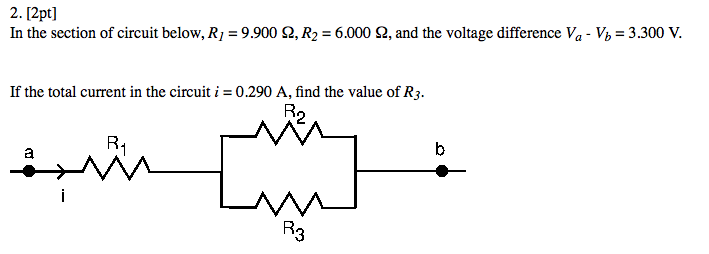In the previous problem, what is the current through R3?

In the section of circuit below, R_1 = 9.900 Ohm, R_2 = 6.000 Ohm, and the voltage difference V_a - V_b = 3.300 V. If the total current in the circuit i = 0.290 A, find the value of R_3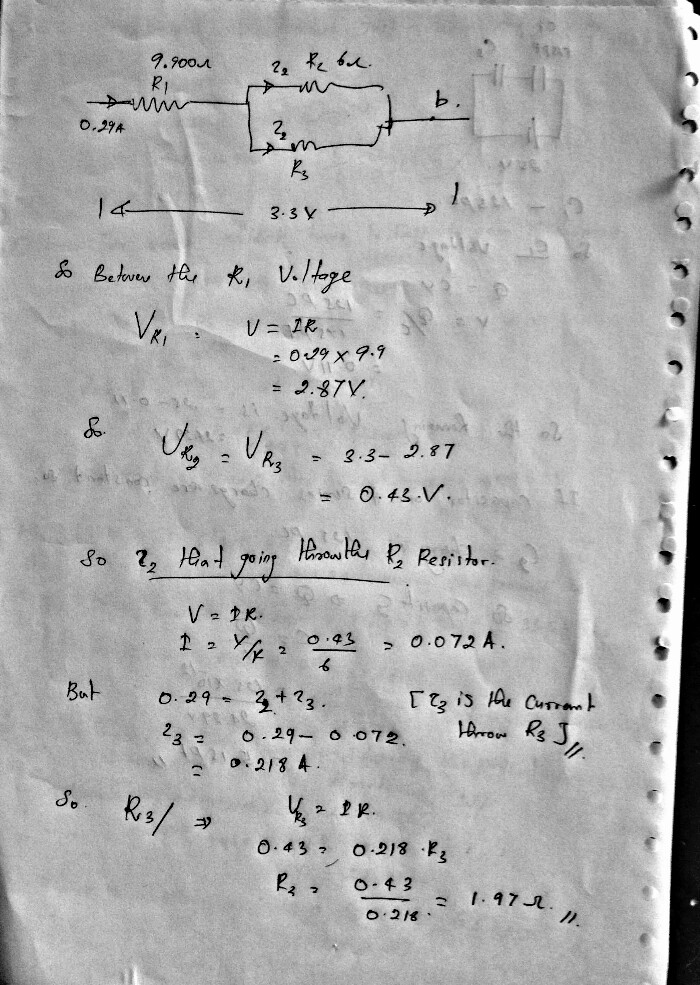#### Earn Coin

Coins can be redeemed for fabulous gifts.

Similar Homework Help Questions
• ### Chapter 27, Problem 21. Find the current through each resistor in the circuit shown in Figure...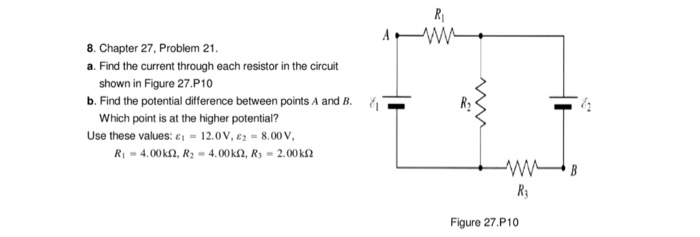Chapter 27, Problem 21. Find the current through each resistor in the circuit shown in Figure 27.P10 Find the potential difference between points A and H. Which point is at the higher potential? Use these values: epsilon_i - 12.0 V, epsilon_2 = 8.00 V. R_1 = 4.00 k Ohm, R_2 = 4.00 k Ohm, R_3 = 2.00 k Ohm

• ### Determine the current through each of the specified points. The circuit to the right consists of...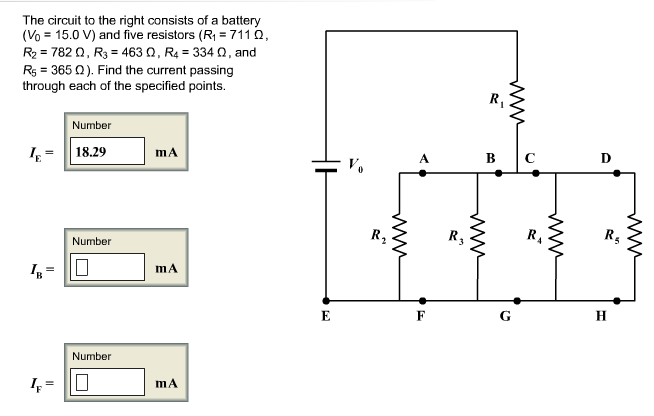Determine the current through each of the specified points. The circuit to the right consists of a battery (V_0 = 15.0 V) and five resistors (R_1 = 711 Ohm, R_2 = 782 Ohm, R_3 = 463 Ohm, R_4 = 334 Ohm, and R_5 = 365 Ohm). Find the current passing through each of the specified points. I_E = 18.29 mA I_B = mA I_F = mA

• ### In the circuit shown below, what is the rate at which energy is dissipated in (a)...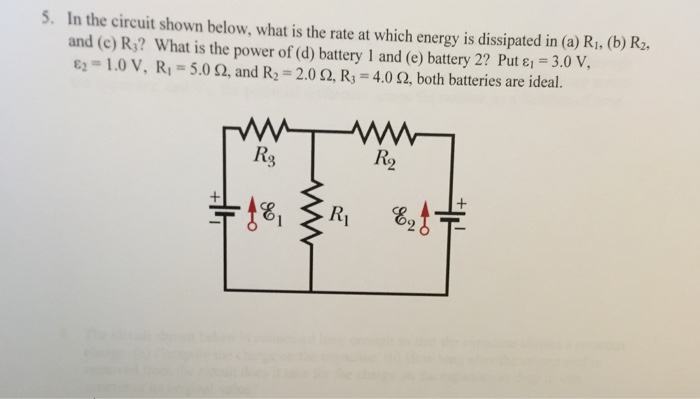In the circuit shown below, what is the rate at which energy is dissipated in (a) R_1, (b) R_2, and (c) R_3? What is the power of (d) battery 1 and (e) battery 2? Put epsilon_1 = 3.0 V, epsilon_2 = 1.0 V, R_1 = 5.0 ohm, and R_2 = 2.0 ohm, R_3 = 4.0 ohm, both batteries are ideal.

• ### I'm in hurry please In the electric circuit in the figure below, epsilon_1 = 9.0 V,...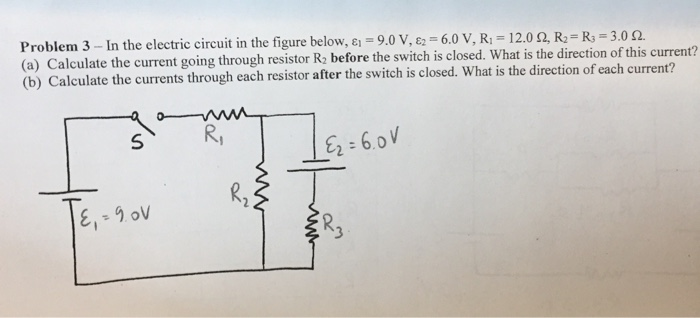I'm in hurry please In the electric circuit in the figure below, epsilon_1 = 9.0 V, epsilon_2 = 6.0V, R_1 = 12.0 ohm, R_2 = R_3 = 3.0 ohm Calculate the current going through resistor R_2 before the switch is closed. What is the direction of this current? Calculate the current through each resistor after the switch is closed. What is the direction of each current?

• ### For the DC circuit shown below, what is the equivalent resistance of the network? What is...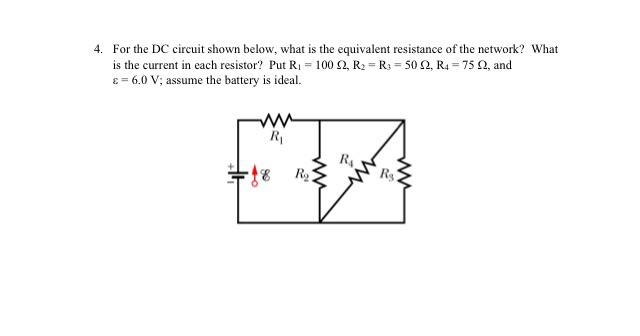For the DC circuit shown below, what is the equivalent resistance of the network? What is the current in each resistor? Put R_1 = 100 Ohm, R_2 = R_3 = 50 Ohm, R_4 = 75 Ohm, and epsilon = 6.0 V; assume the battery is ideal.

• ### Consider the circuit shown below: R_1 = 670 Ohm R_2 = 190 Ohm R_3 = 780...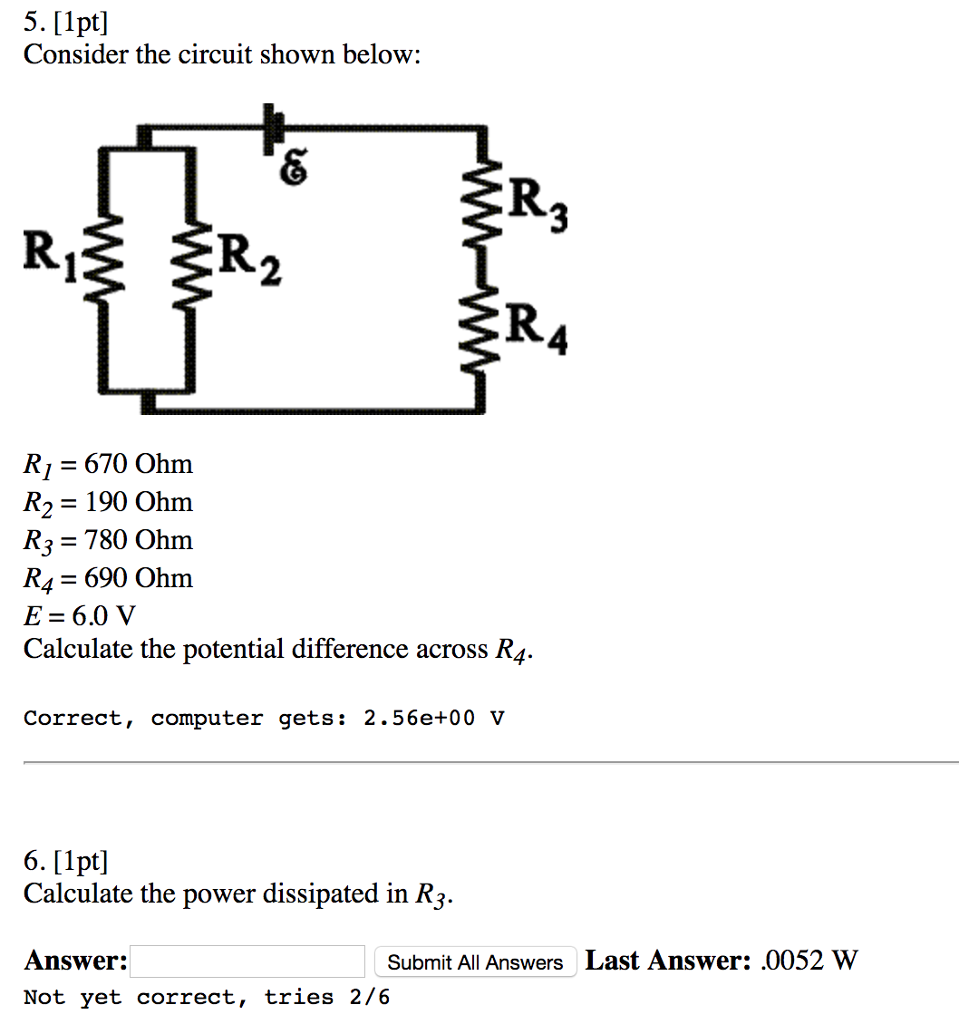Consider the circuit shown below: R_1 = 670 Ohm R_2 = 190 Ohm R_3 = 780 Ohm R_4 = 690 Ohm E = 6.0 V Calculate the potential difference across R_4. Correct, computer gets: 2.56e+00 V Calculate the power dissipated in R_3

• ### Refer to the right figure. Using Kirchhoff's laws, determine the amount of current flow through R_2...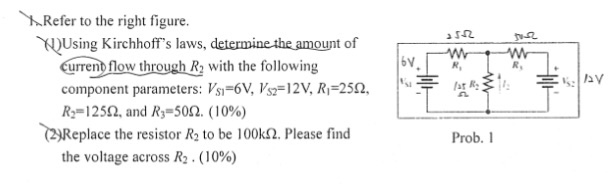Refer to the right figure. Using Kirchhoff's laws, determine the amount of current flow through R_2 with the following component parameters: V_S1 = 6V, V_S2 = 12V, R_1 = 25 Ohm, R_2 = 125 Ohm, and R_3 = 50 Ohm. Replace the resistor R_2 to be 100k Ohm. Please find the voltage across R_2.

• ### In the circuit in the figure below, the batteries have negligible internal resistance. (Take R_1 =...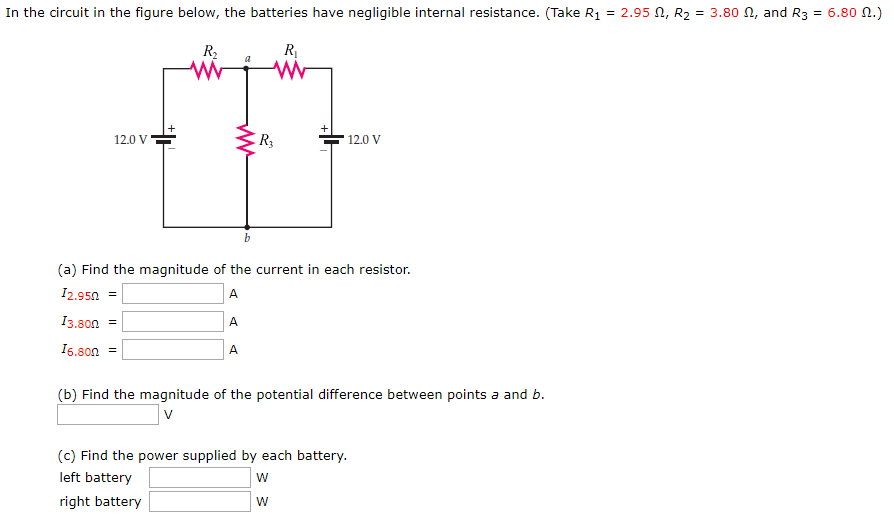In the circuit in the figure below, the batteries have negligible internal resistance. (Take R_1 = 2.95 ohm, R_2 = 3.80 ohm, R_3 = 6.80 ohm.) (a) Find the magnitude of the current in each resistor. I_2.95 ohm = A I_3.80 ohm = A I_6.80 ohm = A (b) Find the magnitude of the potential difference between points a and b. V (c) Find the power supplied by each battery. left battery W right battery W

• ### The emfs and resistances in the circuit shown on the right have the following values: epsilon_1...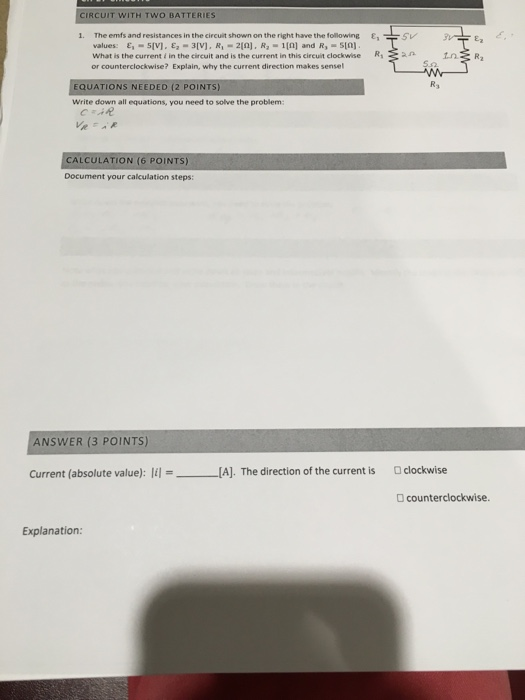The emfs and resistances in the circuit shown on the right have the following values: epsilon_1 = 5[V], epsilon_2 = 3[V], R_1 = 2[Ohm], R_2 = 1[Ohm] and R_3 = 5[Ohm]. What is the current i in the circuit and is the current in this circuit clockwise or counterclockwise? Explain, why the current direction makes sense! Write down all equations, you need to solve the problem: Document your calculation steps: Current (absolute value): [i] = _____ [A]. The direction of...

• ### Consider the circuit shown in the figure below.(Assume R_1 = 11.5 ohm and R_2 = 3.50...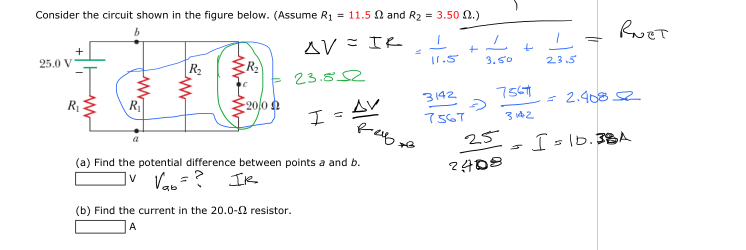Consider the circuit shown in the figure below.(Assume R_1 = 11.5 ohm and R_2 = 3.50 ohm.) v find the potential difference between points a and b. Find the current in the 20.0-ohm resistor. A